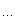< 2022年8月 >
31123456
78910111213
14151617181920
21222324252627
28293031123
45678910

# 随笔档案

•

### 评论排行榜

data, type, newtype

data Mode = ReadMode | WriteMode
data Some = Some Int String
data Thing = { a :: Int, b :: String }
data Func a b = { func :: a -> b }

type IntStringFunc = data Func Int String

type在这里定义了一个别名IntStringFunc类型，包含了一个函数类型是Int -> String的func的数据，这里的type相当于C++ 11的using别名，因为它还可以这样写：

type IntBFunc b = data Func Int b

template <typename T, typename P>
class SomeType;

template <typename T>
using SomeTypeInt = SomeType<T, int>;

newtype NewType a b = NewType { func :: a -> b }

some = Some 0 "test"  -- 定义一个数据，类型为Some

-- 定义两个函数用于获取Some的数据
getSomeInt Some i _ = i
getSomeString Some _ s = s

getSomeInt some  -- 0
getSomeString some  -- "test"

data Maybe a = Nothing
| Just a

useMaybe maybe =
case maybe of
Nothing -> …  -- Maybe的值是空
Just a -> …  -- 直接使用a即可

useMaybe (Just 1)

0 : [1, 2, 3, 4]  -- [0, 1, 2, 3, 4]

useList [] =useList (x:xs) = … -- x是list里面的第一个元素，xs是list的尾部

getTupleValue (_, _, _, (_, _, (x:xs))) = … -- 取得x和xs数据

Visitor模式和C++ template

C++的template meta-programming也可以看成是一种模式匹配，C++里面著名的Factorial求值是这样的：

template <int N>
struct Factorial
{
enum { value = N * Factorial<N - 1>::value };
};

template <>
struct Factorial<0>
{
enum { value = 1 };
};

int v = Factorial<10>::value;

factorial 0 = 1
factorial n = n * factorial (n - 1)

v = factorial 10

C++中的模板参数就是用来做模式匹配的，每特化一种类型就可以匹配某种类型，然后对那种匹配的类型做相应的操作。C++的template meta-programming是编译时期（编译器运行期）的行为，所以它只能操作类型以及编译时期能够确定的值，而模式匹配是程序本身的运行期的行为。

Currying

add :: Int -> (Int -> Int)  -- 一般写成 Int -> Int -> Int
add a b = a + b

Partial application

Currying是一个从左到右部分传参数的一个过程，也就是不会出现参数a还没给，就给了具体的参数b的情况。如果确定要先给参数b，那么它是Partial application，如下：

(+ 2) 1 -- result: 3

(+ 2)这种类似的用法可能会作为参数传递给另外一个函数。Partial application是一种更宽泛的概念，上面的Currying是一种Partial application。

func a b c d e f = …

do1 = func 1
do2 = do1 2
do3 = do2 3
do4 = do3 4
do5 = do4 5

C++: function + bind

C++中的function + bind其实是一种Partial application实现，比如：

int Func(int a, int b, int c);

std::function<int (intint)> f1 = std::bind(Func, 1, std::placeholders::_1, std::placeholders::_2);
std::function<int (int)> f2 = std::bind(f1, std::placeholders::_1, 3);
f2(2); // Func(1, 2, 3);

typeclass

(>>=) :: m a -> (a -> m b) -> m b
(>>) :: m a -> m b -> m b
return :: a -> m a
fail :: String -> m a

data Maybe a = Nothing
| Just a

(>>=) maybeA f =
case maybeA of
Nothing -> Nothing
Just a -> f a

(>>) maybeA maybeB = maybeA >>= (\_ -> maybeB)

return = Just

fail = error

(>>=) :: m a -> (a -> m b) -> m b
(>>) :: m a -> m b -> m b
(>>) ma mb = ma >>= (\_ -> mb)
return :: a -> m a
fail :: String -> m a
fail = error

(>>=) (x:xs) f = (f x) ++ (xs >>= f)
(>>=) [] _ = []
return a = [a]

helloWorld = do
putStr "hello "
putStrLn "world"

helloWorld =
(putStr "hello ") >>
(putStrLn "world")

newtype Parser a = Parser {
runP :: State (ByteString, Context) a

file = repeatP \$ spaceLine <||> normalLine

file就把分析整个C++文件所需的操作都combine到了一起，有了这个分析整个文件的Parser a之后，需要把它跑起来，那就需要定义下面这个函数：

runParse :: Parser a -> ByteString -> (a, (ByteString, Context))
runParse p b = runState (runP p) \$ (b, emptyContext)

newtype StateT s m a = StateT {
runStateT :: s -> m (a, s)
}

class Monad m => MonadState s m | m -> s where
get :: m s
put :: s -> m ()

Lazy evaluation